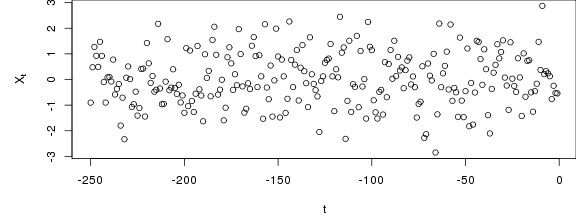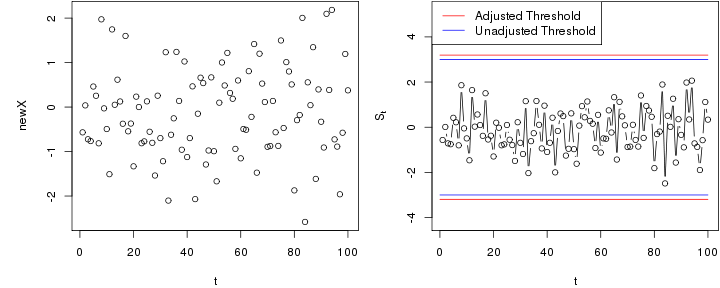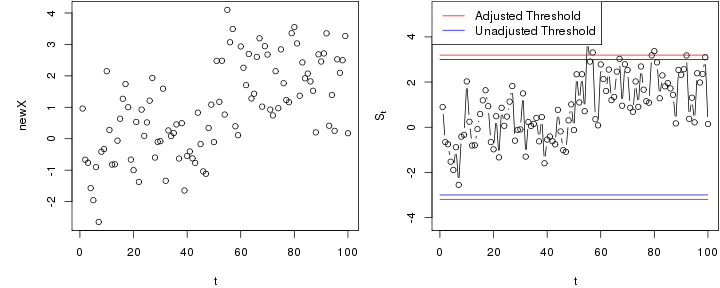# Shewhart chart with estimated in-control state

## Using normality assumptions

Here we consider an application to a two-sided Shewhart chart, assuming that all observations are normally distributed.

Based on $$n$$ past in-control observations $$X_{-n},\dots,X_{-1}$$, the in-control mean can be estimated by $$\hat \mu = \frac{1}{n}\sum_{i=-n}^{-1} X_i$$ and the in-control variance by $$\hat \sigma^2=\frac{1}{n-1}\sum_{i=-n}^{-1} (X_i-\hat \mu)^2$$. For new observations $$X_1,X_2,\dots$$, a two-sided Shewhart chart based on these estimated parameters is defined by $S_t=\frac{X_t-\hat \mu}{\hat \sigma}.$ and signals when $$|S_t|>c$$ for some threshold $$c$$.

The following generates a data set of past observations (replace this with your observed past data).

X <-  rnorm(250)Next, we initialise the chart and compute the estimates needed for running the chart - in this case $$\hat \mu$$ and $$\hat \sigma$$.

library(spcadjust)
chartShew <- new("SPCShew",model=SPCModelNormal(),twosided=TRUE);
xihat <- xiofdata(chartShew,X)
str(xihat)

## List of 3
##  $mu: num 0.0251 ##$ sd: num 1.05
##  \$ m : int 250


## Calibrating the chart to a given average run length (ARL)

We now compute a threshold that with roughly 90% probability results in an average run length of at least 370 in control. This is based on parametric resampling assuming normality of the observations. You should increase the number of bootstrap replications (the argument nrep) for real applications.

cal <- SPCproperty(data=X,nrep=100,
property="calARL", chart=chartShew,
params=list(target=370),quiet=TRUE)
cal

## 90 % CI: A threshold of 3.194 gives an in-control ARL of at least
##   370.
## Based on  100 bootstrap repetitions.


## Run the chart

Next, we run the chart with new observations that are in-control.

newX <- rnorm(100)
S <- runchart(chartShew, newdata=newX,xi=xihat)In the next example, the chart is run with data that are out-of-control from time 51 and onwards.

newX <- rnorm(100,mean=c(rep(0,50),rep(2,50)))
S <- runchart(chartShew, newdata=newX,xi=xihat)